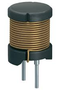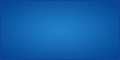## Measure unknown Selfs

A quick and easy way to fairly accurately assess the inductance of an inductor of unknown power.
( provided you have a function generator and an oscilloscope )
Based on an original idea by Ronald DEKKER.

By looking for an easy and inexpensive way to measure chokes ( inductors using the right terms ... ), my favorite search engine that I will not mention here led me after a very short time to website , and then on that of Ronald DEKKER .

I admit that I was very surprised by the simplicity of the method used, and after a few successful tests, I was quickly convinced!

→ However, this technique can only measure inductances between a few tens of µH to a few hundred µH. Below these values, it would be necessary to use a measurement technique using a current generator because of the very low reactance which means that the inductance almost represents a short-circuit in view of the output of the GBF, and on the other hand to exceed these values ​​would require a generator capable of "going up" almost up to 100MHz ...

We all have inductors from all sources ( mainly switching power supplies for me ... ) which once removed from their original printed circuit will fill our drawers. Most of the time, their value is not listed, except for a few exceptions ( Figure 1 ) ...

It may become interesting to know the value of an inductor, for example during the design and / or modification of a power supply, with a view for example to improving the EMC , or even the design of a step-up / step-down converter. voltage.

"... The electromagnetic compatibility ( EMC ) is the ability of a device or system electrical , or electronic, to operate in an electromagnetic environment in a satisfactory manner, without producing itself intolerable electromagnetic disturbances to anything that is in this environment . ... " 3

#### Here is the detail of their method:

Regarding the purely theoretical part, thank you for getting closer to that described by Ronald DEKKER.
Here, no mathematics or complicated calculations, only practice! However, for the more curious, I will still try to explain the principle used at the end of the article.
Everything you need is summarized as follows:

• a generator of functions in sinusoidal mode
• an oscilloscope ( not necessarily Digital ... )
• possibly a frequency meter ( connected to the TTL output of the generator with a BNC / BNC cable type RG58C / U (50 Ω) ... )
• one ( or two ) BNC / BNC type RG58C / U (50 Ω) cable as short as possible, mine measures 45cm
( for example : AMPHENOL - 779829-58-0.6 )
• a BNC type T adapter
( for example : TE CONNECTIVITY / GREENPAR - 5-1634532-1 )
• a male BNC adapter - 2 Female Bananas
( for example : MULTICOMP - SPC20527 )
• a simple calculator

#### The step by step method:

1. Connect the 50 Ω output of the function generator to the oscilloscope using a suitable cable ( see above ), and select a sinusoidal signal.
3. Adjust the generator output voltage to 1 V peak-peak.
4. Adjust the oscilloscope time base to 200 ns / division.
5. Adjust the input level of the CH1 vertical amplifier to 200 mV / div.
( if your screen is graduated like on my HAMEG HM-407, the maximum amplitude of the signal will then be between the 2 levels 0 % and 100 %, i.e. 5 divisions )
6. Connect the unknown inductor in parallel to the CH1 input of the oscilloscope using the BNC-Banana adapter ( Figure 2 ).
WARNING : the connections of the choke to be tested must be as short as possible, which is why my choke is mounted directly on the BNC adapter. Too long and too thin connections risk modifying the total impedance of the RL circuit, and probably also bringing back parasitic capacitances!
The signal will decrease ( maybe even you will hardly see the sinusoidal signal anymore ... ) since this one is directly connected in parallel on CH1.
7. Adjust the input level of the CH1 vertical amplifier to 100 mV / div. ( Figure 4 )
8. Now just adjust the frequency of the generator so that the amplitude on the oscilloscope is exactly half of the original value ( 500 mV c/c, this corresponds to an attenuation of 6 dB ).Figure 3
9. Finally, read the frequency displayed on the generator or the frequency meter ( Figure 5 ), and calculate the value of the inductance according to the : [L = 4,57 / ƒ]. With L in Henry and ƒ in Hz.
You can also prefer L = 4570 / ƒ with L in µH and ƒ in KHz.
10. In the following example, I used a smoothing choke removed from a switching power supply.
If we carry out the above mentioned calculation, that gives us: L = 4570 / 1254 = 3.644338118E0 or 3.644 µH
11. Finally, to check all this with a self ( Figure 3 ) of known value ( marking "221" ), I repeated the procedure, and the result is the following:
the marking "221" means that the inductance is 220 µH. By carrying out the measurement then the calculation as before, the frequency displayed on the generator indicated to me the value of 20,87 KHz, which gives us: L = 4570 / 20,87 = 218,9746047E0 or 218,97 µH
It may not be super precision ( only 0,47 % * of margin of error anyway ... ), but it's already not bad, and it still gives us a fairly good idea. precise value of the inductance to be tested.

Above ( Figures 4 and 5 ) you can see the shape of the original almost sinusoidal signal when testing the 220 µH choke, as well as the frequencies displayed respectively by:

• oscilloscope measurement: 1,26 MHZ
• the measurement displayed by the GBF: 1254,5 KHz
• the measurement displayed by the frequency meter: 1254,457 KHZ

I like to check the accuracy of my measurements ...

#### The theory step by step:

First, let us schematize the circuit ( figure 6 ) ...
The resistance of 50 Ω represents the internal resistance of the generator, itself delimited by the dotted lines. The inductance to be measured is therefore naturally located outside this delimitation. When the generator is "empty", that is to say without load, the internal resistance becomes negligible and the maximum output voltage is therefore found (Vgen = 1Vc / c ).

When connecting the inductance ( L ) to be measured, we are then in the presence of a conventional RL type circuit .
Vscope represents the voltage to be measured by the oscilloscope.
The formula ( figure 7 ) of the designer of this process is as follows:

It may seem complicated like that at first glance, and I admit that I had the same reaction. However, these are only "simplifications" here. To understand the principle of measurement with this equation, I actually took the problem backwards:
I performed the calculations with known values ​​for the inductance as well as for the generator frequency, in order to see where it was going to lead me, and you go and see, it's exceedingly simple!

Let 's proceed in stages:
From my last measure cited in paragraph  11 , I know:

• the value of my inductance: L = 218,97 µH
• the frequency of the generator: ƒ = 20,87 KHz
• the internal resistance of the generator: R = 50 Ω
• the radial pulsation (in rad/s): ω = 2 * π * ƒ
• purely as an indication, I deduce the value of the reactance * of the choke for the frequency ƒ indicated above:
XL = ω * L = 2 * π * ƒ * L = 28,71355304E0 or 28,71 Ω
• and the value of the current by applying Ohm's Law:
I = Vscope / XL = 0,5 / 28,71 = 17,41337964E-3 or 17,41 mA

* in sinusoidal regime, we speak of reactance [Ω] and not of resistance, but this does not change anything when we apply Ohm's Law ...

I carry out my calculation with these known values ​​and it gives me this with the simplified formula ( figure 8 ) either:
[2 * π * ƒ * L] / [√(R² + (2 * π * ƒ) ² * L²] = 497,9959937E-3 which is equivalent to 498 mV, or almost 0,5 V and therefore half of the original voltage of the GBF ( see paragraph  3 )!
→ Thus | Vscop / Vgen | = ½
But it is interesting that ... this corresponds exactly to the value that I am asked to adjust in paragraph 8 in the second phase of the procedure!

Here is why it is necessary to make this essential adjustment, because the rest of the calculations follows directly from it!
And here is this series of calculations ( which I have developed a little for a better understanding ... ) allowing to know the value of an unknown inductance ( figure 9 ). This is why in this second series of equations we calculate the value of the inductance with only half of the original voltage ( 500 mV instead of 1 V ).

The following is really simple, I did it in paragraph 11 , it is enough just to calculate the value of  L according to the following formula:
L(H) = 4,57 / ƒ(Hz)

#### Attention to the small detail:

In fact, during the final simplification, I do not find the value of [4,57], but rather 4,594407462E0 which somewhat distorts the result of the equation developed in figure 9. With this coefficient more precise, I then obtain as an inductance value 220,1441046E-6 which is equivalent to the value of 220.14 µH instead of the 218,97 µH found previously in paragraph 11 . The tolerance is no longer 0.47 % but .
This is why in the calculations I made when writing this article my numbers are represented according to scientific notation. After that, you are free to simplify as you wish by limiting the number of decimal places, knowing that this will inevitably distort your results.

End of the demonstration!

I had indeed announced at the beginning of this article that there would be no mathematical formulas, but I could not give you a ready-made formula, practical for the rest, but not really understandable to all without a minimum additional explanations.

I very much hope that this article has interested you as much as I have been. As usual, I took great pleasure in carrying it out, with the sole aim of transferring knowledge as well as my personal experience.

Philippe.

*Correction note : Thanks to Philippe B. who informed me of an error of decimals which had "slipped" in my explanation of the tolerances.

Source :

### Licence Creative Commons

L'ensemble de ce site hormis une notification spécifique est mis
à  disposition selon les termes de la
Attribution - Pas d'Utilisation Commerciale - Partage dans les Mêmes Conditions
3.0 France.### Mes fournisseurs favorisVérifiez la disponibilité de votre nom de domaine :
 fr com mobi eu net org info name co biz cc tv ws